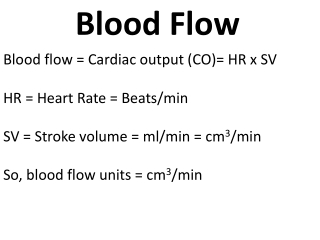DownloadDownload PresentationBlood flow = Cardiac output (CO)= HR x SV HR = Heart Rate = Beats/min

Blood flow = Cardiac output (CO)= HR x SV HR = Heart Rate = Beats/min

Download PresentationBlood flow = Cardiac output (CO)= HR x SV HR = Heart Rate = Beats/min

- - - - - - - - - - - - - - - - - - - - - - - - - - - E N D - - - - - - - - - - - - - - - - - - - - - - - - - - -
Presentation Transcript

1. Blood Flow Blood flow = Cardiac output (CO)= HR x SV HR = Heart Rate = Beats/min SV = Stroke volume = ml/min = cm3/min So, blood flow units = cm3/min

2. Blood Flow = Cardiac Output = • π= 3.14 • η= viscosity = poise • ΔP= pressure gradient = dyne/cm2 • r4= blood vessel average radius = cm4 • l= length = cm • F= Blood Flow = cm3/sec (NOT cm3/min). • Important notes: • To covert blood pressure gradient from mmHg to dyne/cm2 multiply by 1330. • viscosity of plasma = 0.015 poise. Ht=0.45 Males, 0.40 Females.

3. Blood Velocity V= Velocity of blood = cm/sec F= Blood Flow = cm3/sec A= Area of blood vessel = cm2

4. Problem • A male patient has a systolic blood pressure = 160 mmHg and a diastolic blood pressure = 100 mmHg. Calculate the patient’s cardiac output if patient’s body weight = 100 kg, total blood length = 12 m, average blood vessels radius = 0.5 cm . The patient has normal hematocrit value.

5. Answer • 1) Pressure gradient = MAP = diastolic BP + 1/3(systolic BP – diastolic BP) = 100 + 1/3*60= 120 mmHg = 120*1330 = 159600 Dyne/cm2 • l= 12 m = 1200 cm • r4= (0.5)4=0.0625 cm4 • η= 0.015 (1+2.5*0.45)= 0.0318 poise • CO= • CO= 102 cm3/sec = 6118 cm3/min = 6.118 Liters/min approx.

6. Problem 2 • In the same patient, calculate the heart rate if the Stroke volume = 70 cm3/beat.

7. Answer • 2) HR= 6118/70 = 87 beats/min. .

8. Problem • A male patient has a systolic blood pressure = 160 mmHg and a diastolic blood pressure = 100 mmHg. Calculate the patient’s cardiac output if patient’s body weight = 100 kg, total blood length = 12 m, average blood vessels radius = 0.5 cm . The patient has normal hematocrit value. • In the same patient, calculate the heart rate if the Stroke volume = 70 cm3/beat. • If the average blood vessels cross sectional area = 0.3 cm2 , what is the average blood velocity in this patient.

9. Answer • 3) Blood velocity = = 6118/0.3= 20393 cm/min.

10. ده حال كتر القعاد ع الفيسبوك ههههههههههههههههههه كيمبوك